Author: Oscar Cronquist Article last updated on December 17, 2020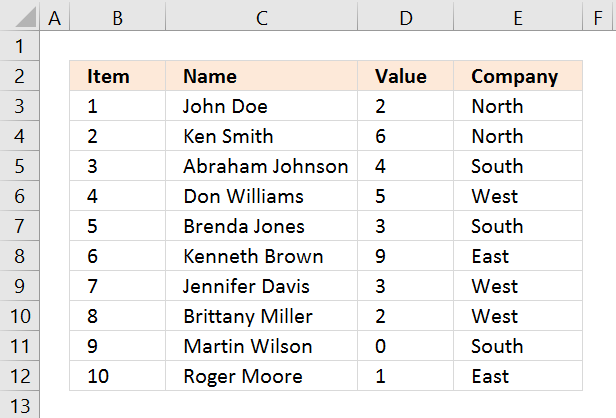I will in this article demonstrate several techniques that extract or filter records based on two conditions applied to a single column in your dataset. For example, if you use the array formula then the result will refresh instantly when you enter new start and end values.

The remaining built-in techniques need a little more manual work in order to apply new conditions, however, they are fast. The downside with the array formula is that it may become slow if you are working with huge amounts of data.

I have also written an article in case you need to find records that match one condition in one column and another condition in another column. The following article shows you how to build a formula that uses an arbitrary number of conditions: Extract records where all criteria match if not empty

This article Extract records between two dates is very similar to the current one you are reading right now, Excel dates are actually numbers formatted as dates in Excel. If you want to search for a text string within a given date range then read this article: Filter records based on a date range and a text string

I must recommend this article if you want to do a wildcard search across all columns in a data set, it also returns all matching records. If you want to extract records based on criteria and not a numerical range then read this part of this article.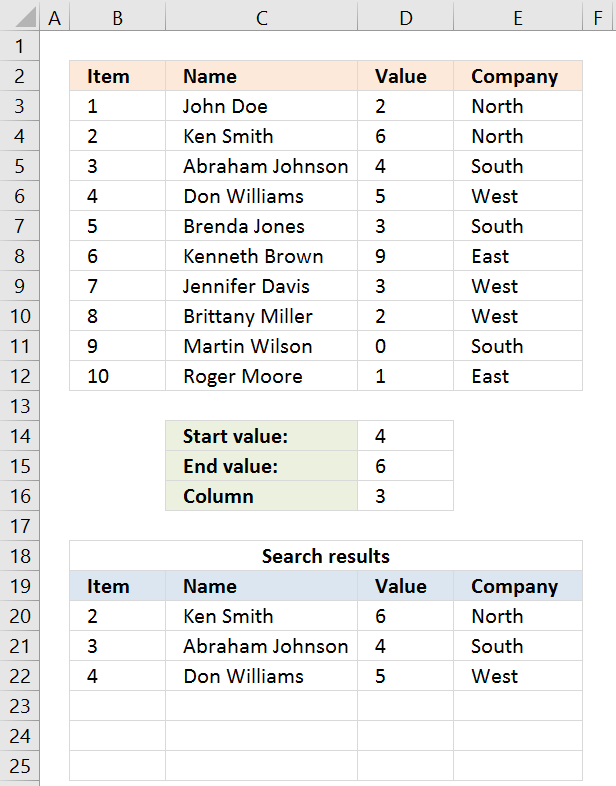The picture above shows you a dataset in cell range B3:E12, the search parameters are in D14:D16. The search results are in B20:E22.

Update 17 December 2020, the new FILTER function is now available for Excel 365 users. Formula in cell B20:

=FILTER(\$B\$3:\$E\$12, (D3:D12<=D15)*(D3:D12>=D14))

It is a regular formula, however, it returns an array of values and extends automatically to cells below and to the right. Microsoft calls this a dynamic array and spilled array.

The array formula below is for earlier Excel versions, it searches for values that meet a range criterion (cell D14 and D15), the formula lets you change the column to search in with cell D16.

This formula can be used with whatever dataset size and shape. To search the first column, type 1 in cell D16.

Update 20 Sep 2017, a smaller formula in cell A20.

Array formula in cell A20:

=INDEX(\$B\$3:\$E\$12, SMALL(IF((INDEX(\$B\$3:\$E\$12, , \$D\$16)< =\$D\$15)*(INDEX(\$B\$3:\$E\$12, , \$D\$16)> =\$D\$14), MATCH(ROW(\$B\$3:\$E\$12), ROW(\$B\$3:\$E\$12)), ""), ROWS(B20:\$B\$20)), COLUMNS(\$A\$1:A1))

See this video to learn more about the formula:

#### How to enter this array formula

1. Select cell A20
2. Paste above formula to cell or formula bar
3. Press and hold CTRL + SHIFT simultaneously
4. Press Enter once
5. Release all keys

The formula bar now shows the formula with a beginning and ending curly bracket, that is if you did the above steps correctly. Like this:

{=array_formula}

Don't enter these characters yourself, they appear automatically.

Now copy cell A20 and paste to cell range A20:E22.

### Explaining array formula in cell A20

You can follow along if you select cell A19, go to tab "Formulas" on the ribbon and click "Evaluate Formula" button.

#### Step 1 - Filter a specific column in cell range \$A\$2:\$D\$11

INDEX(\$B\$3:\$E\$12, , \$D\$16, 1)

becomes

INDEX(\$B\$3:\$E\$12, , 3, 1)

and returns C2:C11

How to use the INDEX function

Gets a value in a specific cell range based on a row and column number.

#### Step 2 - Check which values are in range

(INDEX(\$B\$3:\$E\$12, , \$D\$16, 1)< =\$D\$15)*(INDEX(\$B\$3:\$E\$12, , \$D\$16, 1)> =\$D\$14)

becomes

({2; 6; 4; 5; 3; 9; 3; 2; 0; 1}< =\$C\$14)*({2; 6; 4; 5; 3; 9; 3; 2; 0; 1}> =\$C\$13)

becomes

({2; 6; 4; 5; 3; 9; 3; 2; 0; 1}< =3)*({2; 6; 4; 5; 3; 9; 3; 2; 0; 1}> =0)

becomes

{TRUE; FALSE; FALSE; FALSE; TRUE; FALSE; TRUE; TRUE; TRUE; TRUE}*{TRUE; TRUE; TRUE; TRUE; TRUE; TRUE; TRUE; TRUE; TRUE; TRUE}

and returns

{1; 0; 0; 0; 1; 0; 1; 1; 1; 1}

#### Step 3 - Return corresponding row number

IF((INDEX(\$B\$3:\$E\$12, , \$D\$16)< =\$D\$15)*(INDEX(\$B\$3:\$E\$12, , \$D\$16)> =\$D\$14), MATCH(ROW(\$B\$3:\$E\$12), ROW(\$B\$3:\$E\$12)), "")

becomes

IF({1; 0; 0; 0; 1; 0; 1; 1; 1; 1}, MATCH(ROW(\$B\$3:\$E\$12), ROW(\$B\$3:\$E\$12)), "")

becomes

IF({1; 0; 0; 0; 1; 0; 1; 1; 1; 1}, {1; 2; 3; 4; 5; 6; 7; 8; 9; 10}, "")

and returns

{1; ""; ""; ""; 5; ""; 7; 8; 9; 10}

How to use the IF function

Checks if a logical expression is met. Returns a specific value if TRUE and another specific value if FALSE.

#### Step 4 - Find k-th smallest row number

SMALL(IF((INDEX(\$B\$3:\$E\$12, , \$D\$16)< =\$D\$15)*(INDEX(\$B\$3:\$E\$12, , \$D\$16)> =\$D\$14), MATCH(ROW(\$B\$3:\$E\$12), ROW(\$B\$3:\$E\$12)), ""), ROWS(B20:\$B\$20))

becomes

SMALL({1; ""; ""; ""; 5; ""; 7; 8; 9; 10}, ROWS(B20:\$B\$20))

becomes

SMALL({1; ""; ""; ""; 5; ""; 7; 8; 9; 10}, 1)

and returns 1.

How to use the SMALL function

The SMALL function lets you extract a number in a cell range based on how small it is compared to the other numbers in the group.

#### Step 5 - Return entire row from cell range

INDEX(\$B\$3:\$E\$12, SMALL(IF((INDEX(\$B\$3:\$E\$12, , \$D\$16)< =\$D\$15)*(INDEX(\$B\$3:\$E\$12, , \$D\$16)> =\$D\$14), MATCH(ROW(\$B\$3:\$E\$12), ROW(\$B\$3:\$E\$12)), ""), ROWS(B20:\$B\$20)), COLUMNS(\$A\$1:A1))

becomes

INDEX(\$B\$3:\$E\$12, 1, , 1)

and returns cell range \$B\$3:\$E\$12 in cell range B20:E20: {1,"John Doe",2,"North"}

How to use the INDEX function

Gets a value in a specific cell range based on a row and column number.

Back to top

## Extract all rows from a range that meet criteria in one column [Array formula]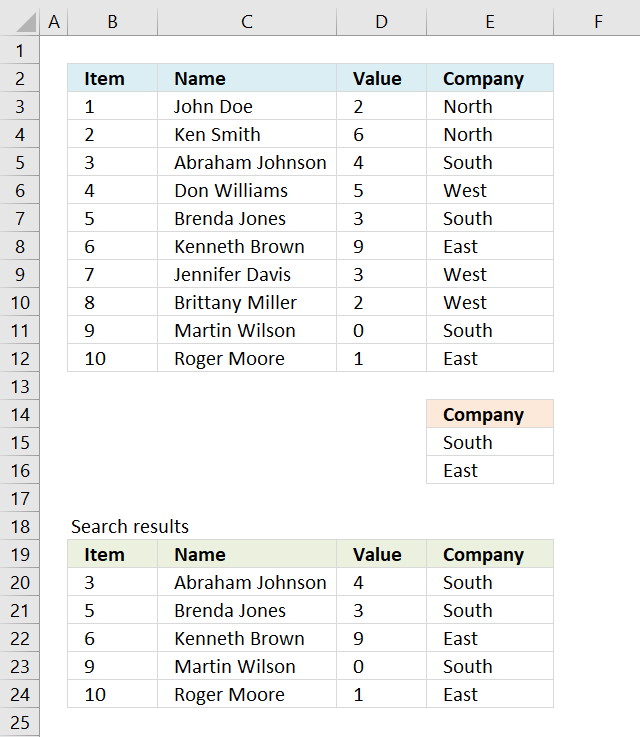The array formula in cell B20 extracts records where column E equals either "South" or "East".

Update 17 December 2020, the new FILTER function is now available for Excel 365 users. Formula in cell B20:

=FILTER(\$B\$3:\$E\$12, COUNTIF(E15:E16, E3:E12))

It is a regular formula, however, it returns an array of values. Read here how it works: Filter values based on criteria

The formula extends automatically to cells below and to the right. Microsoft calls this a dynamic array and spilled array.

The following array formula in cell B20 is for earlier Excel versions:

=INDEX(\$B\$3:\$E\$12, SMALL(IF(COUNTIF(\$E\$15:\$E\$16,\$E\$3:\$E\$12), MATCH(ROW(\$B\$3:\$E\$12), ROW(\$B\$3:\$E\$12)), ""), ROWS(B20:\$B\$20)), COLUMNS(\$B\$2:B2))

To enter an array formula, type the formula in a cell then press and hold CTRL + SHIFT simultaneously, now press Enter once. Release all keys.

The formula bar now shows the formula with a beginning and ending curly bracket telling you that you entered the formula successfully. Don't enter the curly brackets yourself.

### Explaining formula in cell B20

#### Step 1 - Filter a specific column in cell range \$A\$2:\$D\$11

The COUNTIF function allows you to identify cells in range \$E\$3:\$E\$12 that equals \$E\$15:\$E\$16.

COUNTIF(\$E\$15:\$E\$16,\$E\$3:\$E\$12)

becomes

COUNTIF({"South"; "East"},{"North"; "North"; "South"; "West"; "South"; "East"; "West"; "West"; "South"; "East"})

and returns

{0;0;1;0;1;1;0;0;1;1}.

#### Step 2 - Return corresponding row number

The IF function has three arguments, the first one must be a logical expression. If the expression evaluates to TRUE then one thing happens (argument 2) and if FALSE another thing happens (argument 3).

The logical expression was calculated in step 1 , TRUE equals 1 and FALSE equals 0 (zero).

IF(COUNTIF(\$E\$15:\$E\$16,\$E\$3:\$E\$12), MATCH(ROW(\$B\$3:\$E\$12), ROW(\$B\$3:\$E\$12)), "")

becomes

IF({0; 0; 1; 0; 1; 1; 0; 0; 1; 1}, MATCH(ROW(\$B\$3:\$E\$12), ROW(\$B\$3:\$E\$12)), "")

becomes

IF({0; 0; 1; 0; 1; 1; 0; 0; 1; 1}, {1; 2; 3; 4; 5; 6; 7; 8; 9; 10}, "")

and returns

{""; ""; 3; ""; 5; 6; ""; ""; 9; 10}.

#### Step 3 - Find k-th smallest row number

SMALL(IF(COUNTIF(\$E\$15:\$E\$16,\$E\$3:\$E\$12), MATCH(ROW(\$B\$3:\$E\$12), ROW(\$B\$3:\$E\$12)), ""), ROWS(B20:\$B\$20))

becomes

SMALL({""; ""; 3; ""; 5; 6; ""; ""; 9; 10}, ROWS(B20:\$B\$20))

becomes

SMALL({""; ""; 3; ""; 5; 6; ""; ""; 9; 10}, 1)

and returns 3.

#### Step 4 - Return value based on row and column number

The INDEX function returns a value based on a cell reference and column/row numbers.

INDEX(\$B\$3:\$E\$12, SMALL(IF(COUNTIF(\$E\$15:\$E\$16,\$E\$3:\$E\$12), MATCH(ROW(\$B\$3:\$E\$12), ROW(\$B\$3:\$E\$12)), ""), ROWS(B20:\$B\$20)), COLUMNS(\$B\$2:B2))

becomes

INDEX(\$B\$3:\$E\$12, 3, COLUMNS(\$B\$2:B2))

becomes

INDEX(\$B\$3:\$E\$12, 3, 1)

and returns 3 in cell B20.

Back to top

## Extract all rows from a range that meet criteria in one column [Excel defined Table]The image above shows a dataset converted to an Excel defined Table, a number filter has been applied to the third column in the table.

Here are the instructions to create an Excel Table and filter values in column 3.

1. Select a cell in the dataset.
2. Press CTRL + T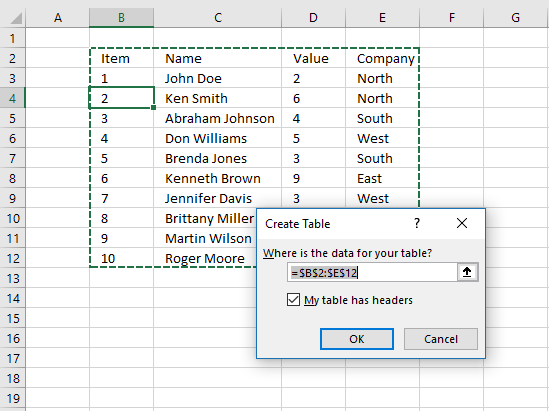3. Click check box "My table has headers".
4. Click OK button.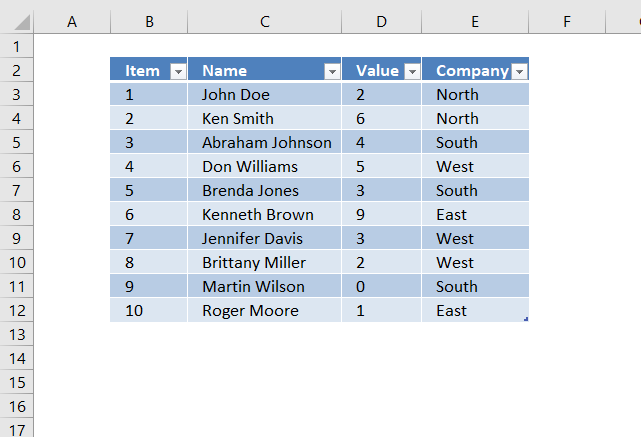The image above shows the Excel defined Table, here is how to filter D between 4 and 6:

1. Click black arrow next to header.
2. Click "Number Filters".
3. Click "Between...".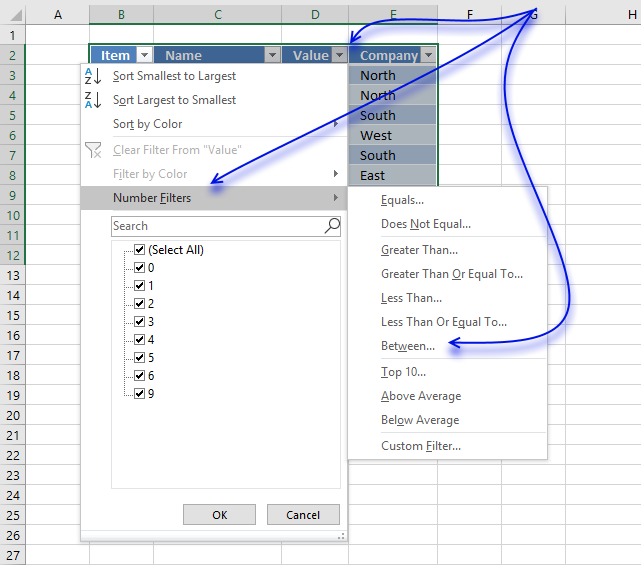4. Type 4 and 6.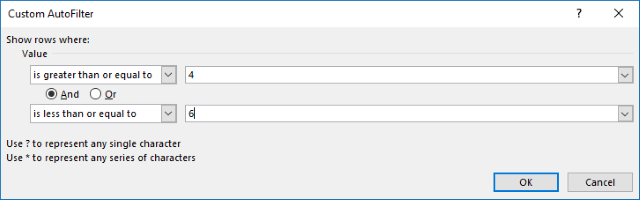5. Click OK button.Back to top

## Extract all rows from a range that meet criteria in one column [Filter]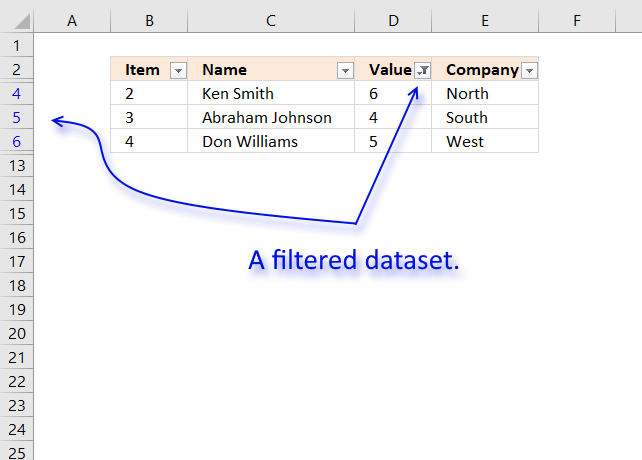The image above shows filtered records based on two conditions, values in column D are larger or equal to 4 or smaller or equal to 6.

Here is how to apply Filter arrows to a dataset.

1. Select any cell within the dataset range.
2. Go to tab "Data" on the ribbon.
3. Click "Filter button".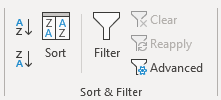Black arrows appear next to each header.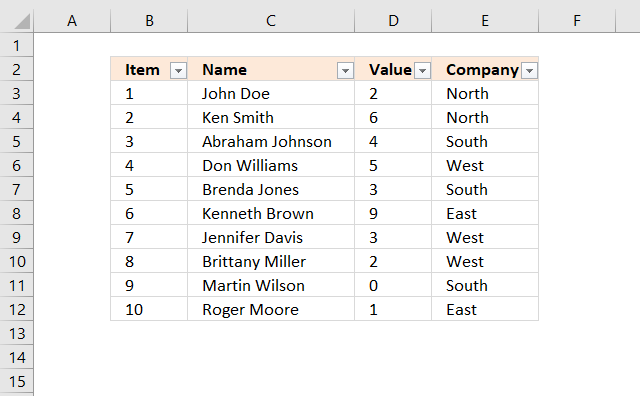Lets filter records based on conditions applied to column D.

1. Click black arrow next to header in Column D, see image below.
2. Click "Number Filters".
3. Click "Between".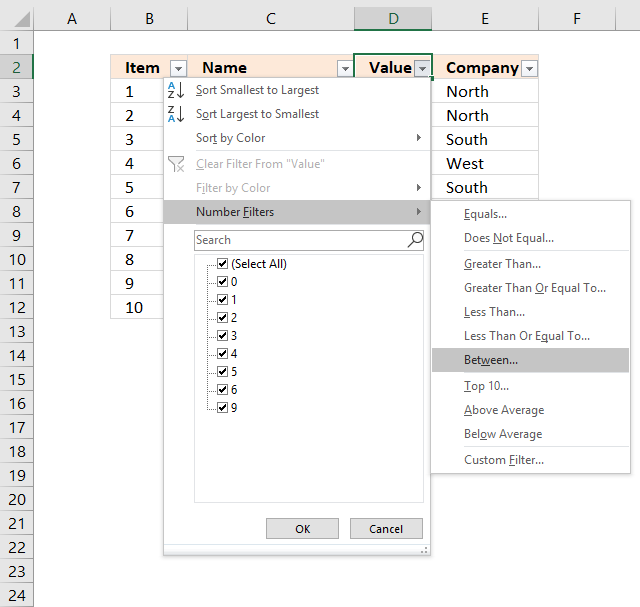4. Type 4 and 6 in the dialog box shown below.5. Click OK button.

Back to top

## Extract all rows from a range that meet criteria in one column [Advanced Filter]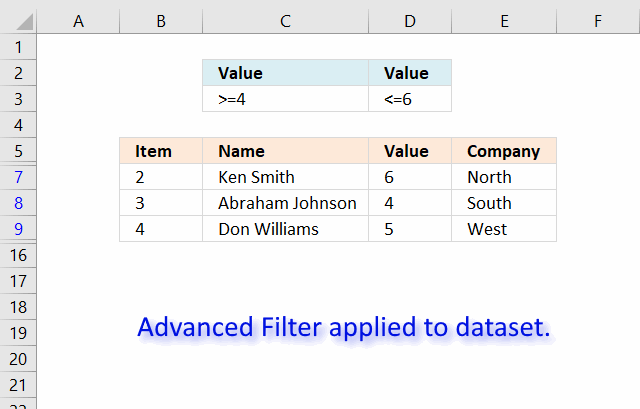The image above shows a filtered dataset in cell range B5:E15 using Advanced Filter which is a powerful feature in Excel.

Here is how to apply a filter:

1. Create headers for the column you want to filter, preferably above or below your data set.
Your filters will possibly disappear if placed next to the data set because rows may become hidden when the filter is applied.
2. Select the entire dataset including headers.
3. Go to tab "Data" on the ribbon.
4. Click the "Advanced" button.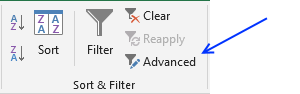5. A dialog box appears.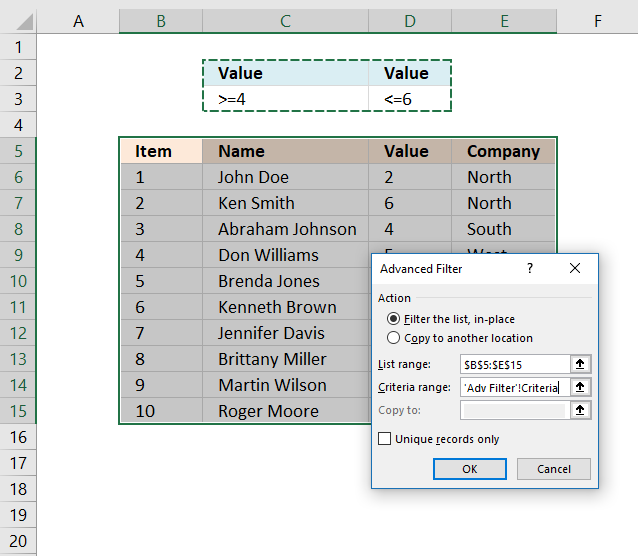6. Select the criteria range C2:D3, shown ithe n above image.
7. Click OK button.Back to top

Back to top

#### Recommended posts

Read this post and see how to extract duplicate records:

Extract duplicate records

This article describes how to filter duplicate rows with the use of a formula.  It is, in fact, an array […]

Learn how to filter unique distinct records:

Filter unique distinct records

Table of contents Filter unique distinct row records Filter unique distinct row records but not blanks Filter unique distinct row […]

Back to top

### Download Excel fileExtract-all-rows-that-contain-a-value-between-this-and-that-part-21v45.xlsx